# Mobile Manipulation Capstone

(Difference between revisions)

This page describes the Capstone Project for the Coursera "Modern Robotics" Specialization. This project forms the sixth and final course: "Modern Robotics, Course 6: Capstone Project, Mobile Manipulation." This project draws on pieces of Courses 1 to 5.

Depending on your programming experience, this project should take approximately 20 hours, broken down into three intermediate milestones and your final submission.

You should use the Modern Robotics code library to help you complete this project.

## Introduction, and the CSV Mobile Manipulation youBot V-REP Scene

In your capstone project, you will write software that plans a trajectory for the end-effector of the youBot mobile manipulator (a mobile base with four mecanum wheels and a 5R robot arm), performs odometry as the chassis moves, and performs feedback control to drive the youBot to pick up a block at a specified location, carry it to a desired location, and put it down.

The final output of your software will be a comma-separated values (csv) text file that specifies the configurations of the chassis and the arm, the angles of the four wheels, and the state of the gripper (open or closed) as a function of time. This specification of the position-controlled youBot will then be "played" on the V-REP simulator to see if your trajectory succeeds in solving the task. This page has information on writing csv files in Python, MATLAB, and Mathematica.

Make sure you have a working V-REP installation (from Course 1 of the Coursera specialization). This project uses Scene 6 (CSV Mobile Manipulation youBot) from the V-REP Introduction wiki page. You should download it and test it with its sample csv file, to see what a solution looks like. (Even if you have downloaded it before, download it again before you begin your project, to make sure you have the most up-to-date version of this scene.) Leave the block's initial and goal configurations as the default. The default initial block configuration is at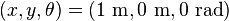$(x,y,\theta) = (1~\text{m}, 0~\text{m}, 0~\text{rad})$ and the final block configuration is at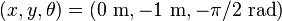$(x,y,\theta) = (0~\text{m},-1~\text{m},-\pi/2~\text{rad})$.

Unlike previous projects, where we used V-REP to simply animate the robot's motion, in this project V-REP will use a physics simulator to simulate the interaction of the youBot with the block. In other words, if the gripper closes on the block in the wrong position or orientation, the block may simply slide out of the grasp. The interaction between the robot and the block is governed by a physics simulator, often called a "physics engine," which approximately accounts for friction, mass, inertial, and other properties. V-REP has different physics engines which you can select, including Bullet and ODE, but ODE is the default for Scene 6.

The time between each successive configuration in your csv file is 0.01 seconds (10 milliseconds). This is an important bit of information, since, unlike the previous visualization scenes which simply animated a csv file with no particular notion of time, the notion of time is critical in a dynamic simulation.

A typical line of your csv file would be something like

-0.75959, -0.47352, 0.058167, 0.80405, -0.91639, -0.011436, 0.054333, 0.00535, 1.506, -1.3338, 1.5582, 1.6136, 0


i.e., thirteen values separated by commas, representing

chassis phi, chassis x, chassis y, J1, J2, J3, J4, J5, W1, W2, W3, W4, gripper state


where J1 to J5 are the arm joint angles and W1 to W4 are the four wheel angles.

Wheels 1 to 4 are numbered as shown in the image to the right. The ten angles (phi for the chassis, five arm joint angles, and four wheel angles) are in radians and the two chassis position coordinates (x,y) are in meters. A gripper state of 0 indicates that you want the gripper to be open, and a gripper state of 1 indicates that you want the gripper to be closed. In practice, the transition from open to closed (or from closed to open) takes up to 0.625 seconds, so any transition from 0 to 1, or 1 to 0, on successive lines in your csv file initiates an action (opening or closing) that will take some time to complete. You should keep the gripper state at the same value for 63 consecutive lines if you want to ensure that the opening/closing operation completes. An opening/closing operation terminates when a force limit is reached (e.g., an object is grasped) or the gripper has fully opened or closed.

Your program will take as input:

• the initial resting configuration of the cube object (which has a known geometry), represented by a frame attached to the center of the object
• the desired final resting configuration of the cube object
• the actual initial configuration of the youBot
• the reference initial configuration of the youBot (which will generally be different from the actual initial configuration, to allow you to test feedback control)
• optionally: gains for your feedback controller (or these gains can be hard-coded in your program)

The output of your program will be:

• a csv file which, when "played" through the V-REP scene, should drive the youBot to successfully pick up the block and put it down at the desired location
• a data file containing the 6-vector end-effector error (the twist that would take the end-effector to the reference end-effector configuration in unit time) as a function of time

Your solution must employ automated planning and control techniques from Modern Robotics. It should not simply be a manually coded trajectory of the robot. Your solution should automatically go from the input to the output, with no other human intervention. In other words, it should automatically produce a working csv file even if the input conditions are changed.

In your software, you should piece together a reference trajectory for the gripper of the robot, which the robot is then controlled to follow. A typical reference trajectory would consist of the following eight segments, as illustrated in the eight images to the right (click on any image to make it larger):

1. A trajectory to move the gripper from its initial configuration to a "standoff" configuration a few cm above the block.
2. A trajectory to move the gripper down to the grasp position.
3. Closing of the gripper.
4. A trajectory to move the gripper back up to the "standoff" configuration.
5. A trajectory to move the gripper to a "standoff" configuration above the final configuration.
6. A trajectory to move the gripper to the final configuration of the object.
7. Opening of the gripper.
8. A trajectory to move the gripper back to the "standoff" configuration.

Segments 3 and 7 each keep the end-effector fixed in space but, at the beginning of the segment, change the state of the gripper from 0 to 1 or 1 to 0, waiting for the gripper closing to complete. In other words, each of these segments would consist of at least 63 identical lines of the csv file (as described above), where the first line of the sequence of identical lines has a gripper state different from the previous line in the csv file, to initiate the opening or closing.

Segments 2, 4, 6, and 8 are simple up or down translations of the gripper of a fixed distance. Good trajectory segments would be cubic or quintic polynomials taking a reasonable amount of time (e.g., one second).

Trajectory segments 1 and 5 are longer motions requiring motion of the chassis. Segment 1 is calculated from the desired initial configuration of the gripper to the first standoff configuration, and segment 5 is calculated from the first standoff configuration to the second standoff configuration. The gripper trajectories could correspond to constant screw motion paths or decoupled Cartesian straight-line motion plus rotational motion, time scaled by third- or fifth-order polynomials (Chapter 9).

Once the entire gripper reference trajectory has been pieced together from the 8 segments, the actual trajectory of the youBot is obtained by using a Jacobian pseudoinverse position controller as described in Chapter 13.5. Starting from the actual initial robot configuration (which has some error from the beginning of reference segment 1), your controller drives the gripper to converge to the reference trajectory. Your feedback controller should eliminate initial error before the gripper attempts to grasp the block, to avoid failure.

To simulate the effect of feedback control, you must write your own motion simulator. For each timestep, you take the initial configuration of the robot and the wheel and joint speeds calculated by your controller and numerically integrate the effect of these speeds over a timestep to get the new robot configuration. To calculate the new configuration of the chassis due to the wheel motions, you must implement an odometry step (Chapter 13.4).

## Kinematics of the youBot

The images to the right illustrate the youBot. Click on them to make them bigger. The description below is consistent with Exercise 13.33 from the book, if you prefer to see the information there. All distances are in meters and all angles are in radians.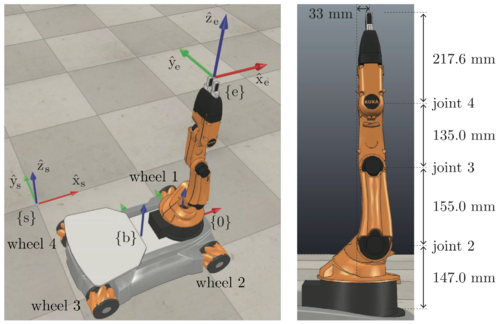This figure illustrates the arm at its home configuration (all joint angles zero) and the frames {s}, {b}, {0}, and {e}. For the image on the right, joint axes 1 and 5 (not shown) point upward and joint axes 2, 3, and 4 are out of the screen. Click to make the image bigger.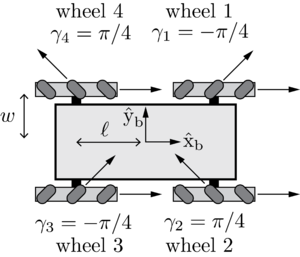A top view of the omnidirectional mobile base. The forward-backward distance between the wheels is 2l = 0.47 meters and the side-to-side distance between wheels is 2w = 0.3 meters. The radius of each wheel is r = 0.0475 meters. The forward driving and "free sliding" direction γ of each wheel is indicated.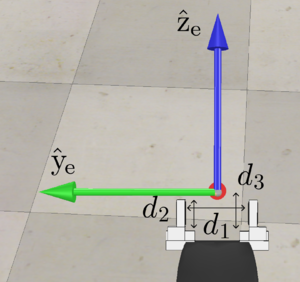The gripper and the end-effector frame {e}, which has an origin midway between the fingers of the gripper. The minimum opening distance of the gripper is d1,min = 2 cm, the maximum opening distance is d1,max = 7 cm, the interior length of the fingers is d2 = 3.5 cm, and the distance from the base of the fingers to the frame {e} is d3 = 4.3 cm. The axis$\hat{\text{x}}_{\text{e}}$ is into the screen.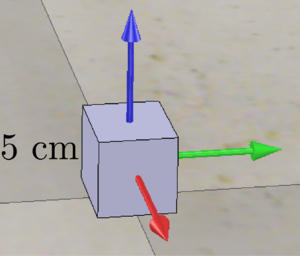The object being manipulated is a cube, 5 cm x 5 cm x 5 cm. The cube's frame {c} is at its center, and the axes are aligned with the edges of the cube.

The configuration of the frame {b} of the mobile base, relative to the frame {s} on the floor, is described by the 3-vector q = (ϕ,x,y) or the SE(3) matrix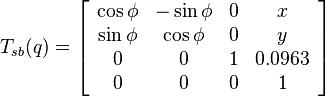$T_{sb}(q) = \left[\begin{array}{cccc} \cos \phi & -\sin \phi & 0 & x \\ \sin \phi & \cos \phi & 0 & y \\ 0 & 0 & 1 & 0.0963 \\ 0 & 0 & 0 & 1 \end{array}\right]$

where z = 0.0963 meters is the height of the {b} frame above the floor. The forward-backward distance between the wheels is 2l = 0.47 meters and the side-to-side distance between wheels is 2w = 0.3 meters. The radius of each wheel is r = 0.0475 meters. The wheel numbering and forward driving and "free sliding" direction γ of each wheel is indicated in the figures.

The fixed offset from the chassis frame {b} to the base frame of the arm {0} is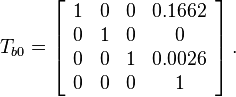$T_{b0}= \left[\begin{array}{cccc} 1 & 0 & 0 & 0.1662 \\ 0 & 1 & 0 & 0 \\ 0 & 0 & 1 & 0.0026 \\ 0 & 0 & 0 & 1 \end{array}\right].$

When the arm is at its home configuration (all joint angles zero, as shown in the figure), the end-effector frame {e} relative to the arm base frame {0} is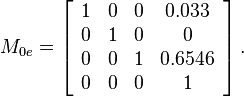$M_{0e}= \left[\begin{array}{cccc} 1 & 0 & 0 & 0.033 \\ 0 & 1 & 0 & 0 \\ 0 & 0 & 1 & 0.6546 \\ 0 & 0 & 0 & 1 \end{array}\right].$

When the arm is at its home configuration, the screw axes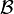$\mathcal{B}$ for the five joints are expressed in the end-effector frame {e} as

1.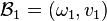$\mathcal{B}_1 = (\omega_1,v_1)$ where$\omega_1 = (0,0,1), \; v_1 = (0,0.033,0)$
2.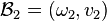$\mathcal{B}_2 = (\omega_2,v_2)$ where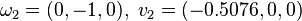$\omega_2 = (0,-1,0), \; v_2 = (-0.5076,0,0)$
3.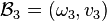$\mathcal{B}_3 = (\omega_3,v_3)$ where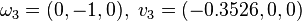$\omega_3 = (0,-1,0), \; v_3 = (-0.3526,0,0)$
4.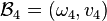$\mathcal{B}_4 = (\omega_4,v_4)$ where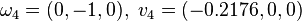$\omega_4 = (0,-1,0), \; v_4 = (-0.2176,0,0)$
5.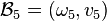$\mathcal{B}_5 = (\omega_5,v_5)$ where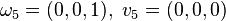$\omega_5 = (0,0,1), \; v_5 = (0,0,0)$

In this project, for simplicity we assume no joint limits on the five joints of the robot arm. It is recommended, however, that you choose limits on the wheel and joint velocities. We will come back to this issue later.

The end-effector frame {e} is rigidly attached to the last link and is midway between the tips of the gripper fingers. The minimum opening distance of the gripper is d1,min = 2 cm (i.e., the fingers cannot fully close; they can only get within 2 cm of each other), the maximum opening distance is d1,max = 7 cm, the interior length of the fingers is d2 = 3.5 cm, and the distance from the base of the fingers to the frame {e} is d3 = 4.3 cm. When the gripper closes, it closes until it reaches its minimum closing distance or encounters a force large enough to prevent further closing.

The object being manipulated is a cube, 5 cm x 5 cm x 5 cm. The cube's frame {c} is at the center of the cube, and the axes are aligned with the edges of the cube. The default initial configuration of the cube is at (x, y, z) = (1, 0, 0.025 ) m in the space frame {s}, and the axes of {c} are aligned with {s}. The default desired final configuration of the cube is at (x, y, z) = (0, -1, 0.025) m and the axes of {c} are rotated by − π / 2 radians about the$\hat{\text{z}}_{\text{s}}$-axis of {s}. These are written in SE(3) as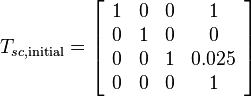$T_{sc,\text{initial}} = \left[\begin{array}{cccc} 1 & 0 & 0 & 1 \\ 0 & 1 & 0 & 0 \\ 0 & 0 & 1 & 0.025 \\ 0 & 0 & 0 & 1\end{array} \right]$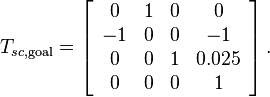$T_{sc,\text{goal}} = \left[\begin{array}{cccc} 0 & 1 & 0 & 0 \\ -1 & 0 & 0 & -1 \\ 0 & 0 & 1 & 0.025 \\ 0 & 0 & 0 & 1\end{array} \right].$

Both the initial and final configurations of the cube can be altered in the V-REP scene. (You can only alter the (x,y) position and the orientation of the cube about the$\hat{\text{z}}_{\text{s}}$-axis.) A good choice for the "standoff" configuration before moving down to the cube and moving back up (the ends of trajectories 1, 4, 5, and 8) is to have the {e} frame a few cm above the {c} frame.

## Some Background on Dynamic Simulations in V-REP

This section contains more information about how the V-REP simulation works. It contains more details than you need for the final project. You may skip this section and go directly to Milestones and Details.

The V-REP scene used for the capstone project (Scene 6, CSV Mobile Manipulation youBot) is the first scene in which we've used V-REP's ability to simulate bodies in contact. A "physics engine" (sometimes called a "game engine," since such simulators are often used in video games) simulates the motions of bodies when forces are applied to them, and when they make contact or collide with each other. Physics engines also handle articulated bodies with joints and other constraints.

V-REP does not have its own physics engine; instead, it bundles and makes available several different physics engines, including Bullet, ODE, Vortex, and Newton. While these simulators have many features in common, they each have their own strengths and weaknesses, and none is perfect. A simulator must detect collisions and contacts, simulate restitution ("bounciness") and friction, employ numerical integration to take timesteps to advance the simulation, etc. All of these are approximate operations with a variety of "magic numbers" and error tolerances, with the goal of making the simulations physically realistic without consuming too much CPU time.

As a result of these approximations, you can often observe bizarre behavior, such as an object spontaneously beginning to bounce on the floor. In this capstone project, the unexpected behavior may be the object slipping out of the grasp. These are just realities of dynamic simulation, and it is beyond the scope of this project to delve into the details of dynamic simulation. If you would like to learn more, you can check out the documentation for ODE and Bullet.

For block grasping, we have found ODE to give the best results, so that is the default physics engine when you open Scene 6.

### Rigid Bodies in Scene 6

In V-REP, each rigid body is classified as either respondable or non-respondable:

• respondable: The body can make contact and collide with other bodies.
• non-respondable: The body can pass through other bodies.

Thus if a respondable body and a non-respondable body overlap, or if two non-respondable bodies overlap, there is no collision. If two respondable bodies make contact, however, forces will keep them from penetrating each other.

In Scene 6, only the floor, the gripper fingers, and the cube are respondable. All other bodies are non-respondable. This means, for example, that the robot can drive over the cube, and the cube will never move. (The chassis and wheels do not interact with the cube.) This also means that the youBot's arm can intersect the mobile base, or arm links can intersect each other. This is unrealistic, of course, but we are focusing on the interaction of the cube with the gripper and the floor.

In addition, each rigid body can be classified as dynamic or non-dynamic:

• dynamic: The body has mass and inertial properties that are used to compute its motion. For example, as shown in the image to the right, you can click on "Cuboid_initial" to learn about the mass properties of the cube in Scene 6. You can also learn about material properties, like "friction values." (The simulated friction coefficient of two bodies in contact is calculated as the product of the friction values of the two materials. This is a simple, but non-physical, way to come up with a friction coefficient for two bodies in contact without having to specify a friction coefficient for every possible pair of materials in contact.)
• non-dynamic: The body's motion is not computed according to dynamics computed by the physics engine.

In Scene 6, only the gripper fingers and the cube are dynamic. In other words, the motions of the fingers and the cube are computed according to the physics engine; other bodies are not part of the dynamic simulation.

### Joints in Scene 6

In Scene 6, each joint is either in torque/force mode or passive mode:

• torque/force mode: In torque/force mode, the motion of the joint is controlled by a torque or force applied by an actuator, possibly governed by a PID control law, for example.
• passive mode: In this mode, the joint is kinematically controlled to go to specified positions, without reference to forces or torques.

In Scene 6, all joints are in passive mode, except for the two joints controlling the gripper motion. All passive joints kinematically follow the motions commanded in the csv file.

All joint limits for the youBot's 5R arm are disabled in Scene 6. This is unrealistic, but respecting joint limits is not part of this capstone project.

### Gripper

The gripper fingers have joint limits: each finger of the gripper can travel 2.5 cm. At maximum opening, the distance between the fingers is 7 cm, and at maximum closing the distance is 2 cm. The gripper fingers are controlled to always be opening (until force limits are reached or the maximum inter-finger distance is obtained) or always closing (until force limits are reached, as when closed on the cube, or the minimum inter-finger distance is obtained).

### Running Your Solution in Scene 6

When you load your csv file into Scene 6 for the first time and press "Play File," the robot will jump to its starting configuration, pause for a second, and then begin executing your csv file. When it jumps like this, the "passive mode" joints are repositioned immediately, but the "torque/force mode" joints (for the gripper fingers) take time to be repositioned. So you may see some weird behavior as the gripper fingers reposition themselves in the initial fraction of a second when you run your csv file the first time. If you don't like this, then just run the csv file a second time. The robot will have been repositioned to the starting point of the csv file before you press "Play File," so the gripper will not have to reposition itself.

## Milestones and Details

Your solution to this project will be a fairly complex piece of software. To help you structure the project, and to allow you to test individual pieces of your solution, the project has three milestones before you finally complete the project. You do not turn in your solutions to these milestone subprojects; you only turn in your final project.

### Milestone 1: youBot Kinematics Simulator and csv Output

You will write a simulator for the kinematics of the youBot. The main function in the simulator, let's call it NextState, is specified by the following inputs and outputs:

Input:

• A 12-vector representing the current configuration of the robot (3 variables for the chassis configuration, 5 variables for the arm configuration, and 4 variables for the wheel angles).
• A 9-vector of controls indicating the arm joint speeds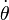$\dot{\theta}$ (5 variables) and the wheel speeds u (4 variables).
• A timestep Δt.
• A positive real value indicating the maximum angular speed of the arm joints and the wheels. For example, if this value is 12.3, the angular speed of the wheels and arm joints is limited to the range [-12.3 radians/s, 12.3 radians/s]. Any speed in the 9-vector of controls that is outside this range will be set to the nearest boundary of the range. If you don't want speed limits, just use a very large number. If you prefer, your function can accept separate speed limits for the wheels and arm joints.

Output: A 12-vector representing the configuration of the robot time Δt later.

The function NextState is based on a simple first-order Euler step, i.e.,

• new arm joint angles = (old arm joint angles) + (joint speeds) * Δt
• new wheel angles = (old wheel angles) + (wheel speeds) * Δt
• new chassis configuration is obtained from odometry, as described in Chapter 13.4

Testing the NextState function: To test your NextState function, you should embed it in a program that takes an initial configuration of the youBot and simulates constant controls for one second. For example, you can set Δt to 0.01 seconds and run a loop that calls NextState 100 times with constant controls$(u,\dot{\theta})$. Your program should write a csv file, where each line has 13 values separated by commas (the 12-vector consisting of 3 chassis configuration variables, the 5 arm joint angles, and the 4 wheel angles, plus a "0" for "gripper open") representing the robot's configuration after each integration step. Then you should load the csv file into the CSV Mobile Manipulation youBot V-REP scene and watch the animation of the constant controls to see if your NextState function is working properly (and to check your ability to produce a csv file).

This page has information on writing csv files in Python, MATLAB, and Mathematica.

Sample controls to try: Simulate the following controls for 1 second and watch the results in the V-REP capstone scene (Scene 6). The controls below are only for the wheels; you can choose the arm joint speeds as you wish.

1. u = (10,10,10,10). The robot chassis should drive forward (in the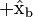$+\hat{\text{x}}_{\text{b}}$ direction) by 0.475 meters.
2. u = ( − 10,10, − 10,10). The robot chassis should slide sideways in the$+\hat{\text{y}}_{\text{b}}$ direction by 0.475 meters.
3. u = ( − 10,10,10, − 10). The robot chassis should spin counterclockwise in place by 1.234 radians.

If the chassis motion is not what is described, then something is wrong with your implementation of odometry. If you are uncertain that your wheel motions and chassis motions correspond to each other, you can check out the five basic mobile base motions shown in a .zip file in the CSV Animation youBot scene.

You should also check that your wheel angles and arm joint angles are being updated properly, too, but this should be easy.

You should also try specifying a speed limit of 5 for the joints and wheels, then try the same tests above. Since your commanded controls exceed the speed limit, your function should properly restrict the actual speeds executed by the wheels and joints to the range [-5, 5]. As a result, the chassis should only move half the distance in these tests.

Review material: This capstone project builds on material throughout the textbook "Modern Robotics: Mechanics, Planning, and Control." Click here for links to the preprint version of the textbook and the videos. Particularly relevant to this milestone are the following chapters and their associated videos:

### Milestone 2: Reference Trajectory Generation

For this milestone you will write a function TrajectoryGenerator to generate the reference trajectory for the end-effector frame {e}. This trajectory consists of eight concatenated trajectory segments, as described above. Each trajectory segment begins and ends at rest.

Input:

• The initial configuration of the end-effector in the reference trajectory: Tse,initial.
• The cube's initial configuration: Tsc,initial.
• The cube's desired final configuration: Tsc,final.
• The end-effector's configuration relative to the cube when it is grasping the cube: Tce,grasp.
• The end-effector's standoff configuration above the cube, before and after grasping, relative to the cube: Tce,standoff. This specifies the configuration of the end-effector {e} relative to the cube frame {c} before lowering to the grasp configuration Tce,grasp, for example.
• The number of trajectory reference configurations per 0.01 seconds: k. The value k is an integer with a value of 1 or greater. Although your final animation will be based on snapshots separated by 0.01 seconds in time, the points of your reference trajectory (and your controller servo cycle) can be at a higher frequency. For example, if you want your controller to operate at 1000 Hz, you should choose k = 10 (10 reference configurations, and 10 feedback servo cycles, per 0.01 seconds). It is fine to choose k = 1 if you'd like to keep things simple.

Outputs:

• A representation of the N configurations of the end-effector along the entire concatenated eight-segment reference trajectory. Each of these N reference points represents a transformation matrix Tse of the end-effector frame {e} relative to {s} at an instant in time, plus the gripper state (0 or 1). For example, if your entire eight-segment trajectory takes 30 seconds, for example, you will have approximately$N \approx 30k/0.01$ sequential reference configurations (perhaps one or a few more, depending on how you treat boundary conditions), each separated by 0.01 / k seconds in time. These reference configurations will be used by your controller. Your representation of the reference configurations could be anything you want. If you use an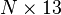$N \times 13$ matrix, for example, each of the N rows would represent a configuration of the end-effector frame {e} relative to {s} at that instant in time. Twelve of the 13 entries of a matrix row are the top three rows of the transformation matrix Tse at that instant of time, i.e., r11,r12,r13,r21,r22,r23,r31,r32,r33,px,py,pz from$T_{se} = \left[\begin{array}{cccc} r_{11} & r_{12} & r_{13} & p_x \\ r_{21} & r_{22} & r_{23} & p_y \\ r_{31} & r_{32} & r_{33} & p_z \\ 0 & 0 & 0 & 1 \end{array}\right],$

and the 13th entry is the gripper state: 0 = open, 1 = closed. Keep in mind that opening and closing the gripper takes up to 1 second (initiated when the gripper state transitions from 0 to 1, or 1 to 0, in your csv file), so the trajectories involving opening and closing the gripper should keep the {e} frame stationary while the gripper completes its motion.

• A csv file with the entire eight-segment reference trajectory. Each line of the csv file corresponds to one configuration Tse of the end-effector, expressed as 13 variables separated by commas. The 13 variables are, in order,
r11, r12, r13, r21, r22, r23, r31, r32, r33, px, py, pz, gripper state


It is up to you to determine the duration of each trajectory segment, but it is recommended that each segment's duration be an integer multiple of 0.01 seconds. You could automatically choose the duration of each trajectory segment to be equal to the maximum of: the distance the origin of the {e} frame has to travel divided by some reasonable maximum linear velocity of the end-effector, and the angle the {e} frame must rotate divided by some reasonable maximum angular velocity of the end-effector. The duration of each trajectory should not be so short as to require unreasonable joint and wheel speeds.

Your TrajectoryGenerator function is likely to use either ScrewTrajectory or CartesianTrajectory, from the Modern Robotics code library, to generate the individual trajectory segments.

Testing your function: We have created a V-REP scene (Scene 8) to help you test your TrajectoryGenerator function. This scene reads in your csv file and animates it, showing how the end-effector frame moves as a function of time. You should verify that your TrajectoryGenerator works as you expect before moving on with the project. This video shows an example of a typical output of your trajectory generator function, as animated by V-REP Scene 8.

Review material: This capstone project builds on material throughout the textbook "Modern Robotics: Mechanics, Planning, and Control." Click here for links to the preprint version of the textbook and the videos. Particularly relevant to this milestone are the following chapters and their associated videos:

### Milestone 3: Feedforward Control

Now that you are able to simulate the motion of the robot and generate a reference trajectory for the end-effector, you are ready to begin experimenting with feedback control of the mobile manipulator. You will write the function FeedbackControl to calculate the kinematic task-space feedforward plus feedback control law, written both as Equation (11.16) and (13.37) in the textbook: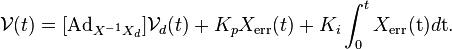$\mathcal{V}(t) = [\text{Ad}_{X^{-1} X_d}] \mathcal{V}_d(t) + K_p X_{\text{err}}(t) + K_i \int_0^t X_{\text{err}}(\text{t}) d\text{t}.$

Input:

• The current actual end-effector configuration X (also written Tse).
• The current end-effector reference configuration Xd (i.e., Tse,d).
• The end-effector reference configuration at the next timestep in the reference trajectory, Xd,next (i.e., Tse,d,next), at a time Δt later.
• The PI gain matrices Kp and Ki.
• The timestep Δt between reference trajectory configurations.

Output:

• The commanded end-effector twist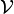$\mathcal{V}$ expressed in the end-effector frame {e}.

To calculate the control law FeedbackControl, we need the current actual end-effector configuration X(q,θ), a function of the chassis configuration q and the arm configuration θ. The values (q,θ) come directly from the simulation results (Milestone 1). In other words, assume perfect sensors.

The error twist Xerr that takes X to Xd in unit time is extracted from the 4x4 se(3) matrix [Xerr] = log(X − 1Xd). FeedbackControl also needs to maintain an estimate of the integral of the error, e.g., by adding XerrΔt to a running total at each timestep. The feedforward reference twist$\mathcal{V}_d$ that takes Xd to Xd,next in time Δt is extracted from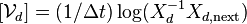$[\mathcal{V}_d] = (1/\Delta t) \log(X_{d}^{-1} X_{d,\text{next}})$. (Make sure you understand why the factor (1 / Δt) is there!)

The output of FeedbackControl is the commanded end-effector twist$\mathcal{V}$ expressed in the end-effector frame {e}. To turn this into commanded wheel and arm joint speeds$(u,\dot{\theta})$, we use the pseudoinverse of the mobile manipulator Jacobian Je(θ),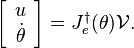$\left[\begin{array}{c} u \\ \dot{\theta} \end{array} \right] = J_e^\dagger(\theta) \mathcal{V}.$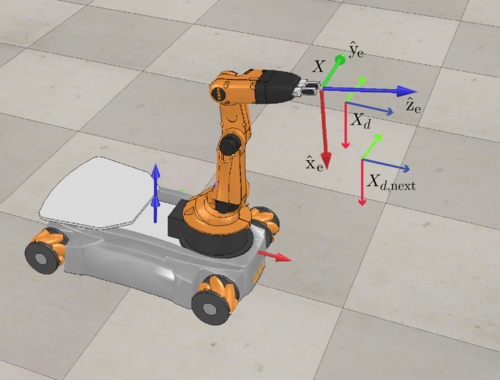This figure illustrates a test for your FeedbackControl and Jacobian pseudoinverse functions. The current robot configuration (q,θ) is shown, as well as the current end-effector configuration X, the current reference end-effector configuration Xd, and the reference end-effector configuration Xd,next a time Δt later.

Testing your FeedbackControl function and your Jacobian pseudoinverse: Before moving on, it is a good idea to confirm that you are correctly calculating$\mathcal{V}$ and the controls$(u,\dot{\theta})$ using the Jacobian pseudoinverse. Here are some test inputs you should try:

• robot configuration: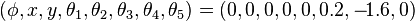$(\phi, x, y, \theta_1, \theta_2, \theta_3, \theta_4, \theta_5) = (0, 0, 0, 0, 0, 0.2, -\!1.6, 0)$, as illustrated in the figure to the right. In other words, all configuration variables are zero except arm joint angle 3, which is 0.2 radians, and arm joint angle 4, which is -1.6 radians.
•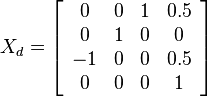$X_d = \left[\begin{array}{cccc} 0 & 0 & 1 & 0.5 \\ 0 & 1 & 0 & 0 \\ -1 & 0 & 0 & 0.5 \\ 0 & 0 & 0 & 1\end{array}\right]$
•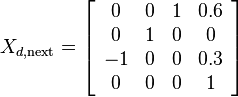$X_{d,\text{next}} = \left[\begin{array}{cccc} 0 & 0 & 1 & 0.6 \\ 0 & 1 & 0 & 0 \\ -1 & 0 & 0 & 0.3 \\ 0 & 0 & 0 & 1\end{array}\right]$
•$X = \left[\begin{array}{cccc} 0.170 & 0 & 0.985 & 0.387 \\ 0 & 1 & 0 & 0 \\ -0.985 & 0 & 0.170 & 0.570 \\ 0 & 0 & 0 & 1\end{array}\right]$ (this is calculated from the robot configuration given above)
• Kp and Ki matrices are zero matrices
• Δt = 0.01

With these inputs, your program should give you

•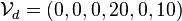$\mathcal{V}_d = (0,0,0,20,0,10)$
•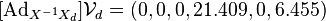$[\text{Ad}_{X^{-1} X_d}] \mathcal{V}_d = (0,0,0,21.409,0,6.455)$
•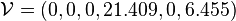$\mathcal{V} = (0,0,0,21.409,0,6.455)$
•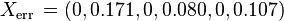$X_{\text{err}} \, = (0,0.171,0,0.080,0,0.107)$
• increment to the numerical integral of the error should be Xerr * Δt
•$J_e = \left[\begin{array}{ccccccccc} 0.030 & -0.030 & -0.030 & 0.030 & -0.985 & 0 & 0 & 0 & 0 \\ 0 & 0 & 0 & 0 & 0 & -1 & -1 & -1 & 0 \\ -0.005 & 0.005 & 0.005 & -0.005 & 0.170 & 0 & 0 & 0 & 1 \\ 0.002 & 0.002 & 0.002 & 0.002 & 0 & -0.240 & -0.214 & -0.218 & 0 \\ -0.024 & 0.024 & 0 & 0 & 0.221 & 0 & 0 & 0 & 0 \\ 0.012 & 0.012 & 0.012 & 0.012 & 0 & -0.288 & -0.135 & 0 & 0 \end{array}\right]$
•$(u,\dot{\theta}) = (157.2,157.2,157.2,157.2,0,-652.9,1398.6,-745.7,0)$

Looking at the figure, these results should make sense to you. The feedforward twist$\mathcal{V}_d$, from Xd to Xd,next, should only have nonzero linear components, since the orientation of both frames is the same; these linear components should be in the x and z directions of the Xd frame; and the component in the x direction should be larger. Expressing this twist in the current frame of the end-effector at X,$[\text{Ad}_{X^{-1} X_d}] \mathcal{V}_d$, shows us that there are still only x and z components, but the component in the X frame's x direction is a bit larger and the component in the z direction is a bit smaller. Since the feedback gains are zero, the commanded twist$\mathcal{V}$ is just$[\text{Ad}_{X^{-1} X_d}] \mathcal{V}_d$. The error Xerr (from X to Xd) shows us that, to drive X to Xd, we must rotate in the positive direction about$\hat{\text{y}}_{\text{e}}$ and move linearly in the$\hat{\text{x}}_{\text{e}}$ and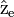$\hat{\text{z}}_{\text{e}}$ directions. Finally, the calculated controls u drive the wheels forward at equal speed (so the chassis translates forward) and only arm joints 2, 3, and 4 rotate, since joints 1 and 5 do not help generate$\mathcal{V}$. Note: The joint speeds in this illustrative example are unreasonably large!

If your Kp matrix is the identity matrix instead of zero, then you should get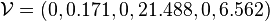$\mathcal{V} = (0,0.171,0,21.488,0,6.562)$ and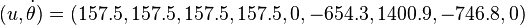$(u,\dot{\theta}) = (157.5,157.5,157.5,157.5,0,-654.3,1400.9,-746.8,0)$.

If you don't get these results, you should correct your program before moving on.

Singularities: If the 6x9 Jacobian matrix Je is singular (i.e., its rank drops below 6) or nearly singular, the pseudoinverse algorithm may generate a pseudoinverse$J_e^\dagger$ with unreasonably large entries, which could lead to unacceptably large commanded joint speeds if we ask for even a very small component of a twist in a direction that the near-singularity nearly prevents. A better behavior for the robot would be to essentially ignore any requested twist components in directions that the near-singularity renders difficult to achieve. To achieve this in MATLAB and Mathematica, you may wish to explore the tolerance options for the pseudoinverse algorithm. The tolerance option allows you to specify how close to zero a singular value must be to be treated as zero. By treating small singular values (that are greater than the default tolerance) as zero, you will avoid having pseudoinverse matrices with unreasonably large entries. A MATLAB example is shown below:

>> M = [[2,0];[0,0.00001]]   % a matrix that's nearly singular
M =
2.000000          0
0   0.000010

>> s = svd(M)  % confirming that the singular values are 2 and 1e-5, i.e., the 2nd singular value is very small
s =
2.000000
0.000010

>> Mp = pinv(M)  % the default MATLAB pseudoinverse treats the 2nd singular value as nonzero; Mp has nonzero entries of 0.5 and 100,000
Mp =
1.0e+05 *
0.000005          0
0   1.000000

>> Mptol = pinv(M,1e-4)  % tell pinv to treat singular values less than 0.0001 as zero; Mptol has no large entries
Mptol =
0.500000          0
0          0


(You may find it better to use an even larger tolerance than 1e-4, e.g., 1e-3 or 1e-2. Experiment!) In any case, you should always place "reasonable" limits on the maximum speeds for the robot joints and wheels, to mimic the limitations of a real robot and to prevent the simulated robot from moving wildly. Other methods have been proposed in the robotics literature to deal with the nearly-singular-Jacobian issue. Feel free to experiment with other methods if you wish.

Why does the robot arm always seem to approach a singularity? You may notice that your controller tends to make the robot arm approach a singularity (e.g., straighten out) before the wheels move much to help move the end-effector. Why do you think this is? Think about the properties of the pseudoinverse. How does it extract a single solution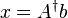$x = A^\dagger b$ to the equation Ax = b when there are many solutions x? How would things change if the wheels had a much larger radius?

Implementing joint limits to avoid self-collisions and singularities (optional, but recommended): Until now, we have not implemented joint limits, so you can easily command robot configurations that result in self-collision, i.e., the arm links intersect each other or the mobile base. You can also command the robot arm to come close to a singularity, e.g., by making the angles of joints 3 and 4 zero (or nearly zero). Singularities like this can cause a problem when you are tracking trajectories specified in terms of the motion of the end-effector (see the discussion above).

To avoid these problems, you can implement joint limits. For example, you could constrain joints 3 and 4 to always be less than − 0.2 radians (or so). The arm will avoid singularities occurring when joints 3 or 4 are at the zero angle, but it will still be able to perform many useful tasks, such as the block pick-and-place task of this capstone. To avoid self-collisions, you can use the arm joint angle sliders in Scene 3: Interactive youBot to approximately find the joint-angle combinations that avoid self-collision. Your approximation should be conservative, meaning that allowed configurations are never in self-collision. But it should not be so conservative that the arm's workspace is overly constrained, preventing the robot from doing useful work.

With these joint limits, you could write a function called testJointLimits to return a list of joint limits that are violated given the robot arm's configuration θ.

You should make sure that your robot's initial configuration satisfies all the joint limits. Then, each time you calculate your wheel and arm joint speeds$(u,\dot{\theta})$ using the pseudoinverse$J_e^\dagger$, use testJointLimits to check if the new configuration at a time Δt later will violate the joint limits. If so, you should recalculate the controls$(u,\dot{\theta})$, by first changing the Jacobian Je to indicate that the offending joint(s) should not be used---the robot must use other joints (if possible) to generate the desired end-effector twist$\mathcal{V}$. To recalculate the controls, change each column of Je corresponding to an offending joint to all zeros. This indicates that moving these joints causes no motion at the end-effector, so the pseudoinverse solution will not request any motion from these joints.

This method is simple, but there are other ways to avoid joint limits. If you use a different approach, be sure to document it in your final README file.

Tip from a Coursera student! "Try making a version that avoids joint limits and self-collisions! I recommend making a new function other than testJointLimits to see the difference between your original code and the enhanced code. Use the original code to find a trajectory which causes the robot to self collide and then use the enhanced one. It's pretty easy and fun to watch."

The full program: Now write your full program, according to the input specifications at the top of this page. Your program should first generate a reference trajectory using TrajectoryGenerator and set the initial robot configuration, a 13-vector as described earlier on this page:

chassis phi, chassis x, chassis y, J1, J2, J3, J4, J5, W1, W2, W3, W4, gripper state


Now the program enters a loop that loops through the reference trajectory generated by TrajectoryGenerator. If the reference trajectory has N reference configurations, the loop runs N − 1 times. For example, the 10th time through the loop, the controller uses the 10th configuration of the reference trajectory as Xd and the 11th configuration as Xd,next to calculate the feedforward twist$\mathcal{V}_d$.

Each time through the loop, you

• calculate the control law using FeedbackControl and generate the wheel and joint controls using$J_e^\dagger(\theta)$;
• send the controls, configuration, and timestep to NextState to calculate the new configuration;
• store every kth configuration for later animation (note that the reference trajectory has k reference configurations per 0.01 second step, as described in Milestone 2; you may choose k = 1 for simplicity); and
• store every kth Xerr 6-vector, so you can later plot the evolution of the error over time.

Once the program has completed all iterations of the loop, it should write out the csv file of configurations. If the total time of motion of the youBot is 15 seconds, your csv file should have 1500 lines (or 1501 lines), corresponding to 0.01 seconds between each configuration. Load the csv file into the CSV Mobile Manipulation youBot scene (Scene 6) to see the results. Your program should also generate a file with the log of the Xerr 6-vector as a function of time, suitable for plotting by your favorite plotting software.

Testing feedforward control: You should make sure feedforward control works as you expect before testing feedback control. Choose an initial configuration of the robot that puts the end-effector exactly at the configuration at the beginning of the reference trajectory. Run your program with Kp = Ki = 0, i.e., feedforward control only. This should result in a csv file which, when played through the V-REP capstone scene, drives the robot to pick up the block and put it down at the desired configuration. (Or at least it should come very close to doing so! Small numerical error in the integration will be fixed when you add a feedback controller.) If not, time to start debugging! Your end-effector reference trajectory must be correct, if you already tested Milestone 2. So now you have to figure out why the wheel and arm controls your feedforward controller and Jacobian pseudoinverse are generating do not drive the end-effector along the reference trajectory. (You could try removing joint speed limits if these are preventing the robot from following the planned trajectory, or increase the time of the trajectory so large joint speeds are not needed to follow the trajectory.)

You should also try starting the end-effector with some initial error from the reference trajectory, but still only use feedforward control. See how the end-effector moves under these circumstances. Does it make sense to you?

Do not move on with the project until your feedforward control works as you expect. Otherwise the effects of PI feedback control will only further confuse the situation.

The physics engine in V-REP: By default, Scene 6 (the capstone mobile manipulation scene) uses a simulation timestep of dt = 10 ms and the physics engine ODE. You should keep the timestep at 10 ms for simulated time to be correct, and we have found ODE to yield more easily understood results than Bullet for Scene 6. But you are welcome to try different physics engines if you'd like; specify your choice in the V-REP GUI. Keep in mind that simulation of bodies in contact is computationally intensive, and approximate solution methods could lead to unexpected behavior, like the block slipping in the grasp. We don't have a suggested "fix" for this; if you want to learn about how physics engines work, and the various approximations they make, you are encouraged to consult the documentation for ODE and Bullet.

Review material: This capstone project builds on material throughout the textbook "Modern Robotics: Mechanics, Planning, and Control." Click here for links to the preprint version of the textbook and the videos. Particularly relevant to this milestone are the following chapters and their associated videos:

### Final Step: Completing the Project and Your Submission

Now that feedforward control is working, you are ready to complete your project. Use the default initial and goal configurations for the cube in the capstone V-REP scene, i.e., the initial cube configuration is at$(x,y,\theta) = (1~\text{m}, 0~\text{m}, 0~\text{rad})$ and the final cube configuration is at$(x,y,\theta) = (0~\text{m},-1~\text{m},-\pi/2~\text{rad})$. Let the initial configuration of the end-effector reference trajectory be at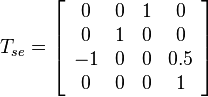$T_{se} = \left[\begin{array}{cccc} 0 & 0 & 1 & 0 \\ 0 & 1 & 0 & 0 \\ -1 & 0 & 0 & 0.5 \\ 0 & 0 & 0 & 1 \end{array}\right]$

Choose an initial configuration of the youBot so that the end-effector has at least 30 degrees of orientation error and 0.2 m of position error. Try executing the feedforward controller (Kp = Ki = 0) and play the resulting csv file through the V-REP capstone scene to see what happens.

Now add a positive-definite diagonal proportional gain matrix Kp while keeping the integral gains zero. You can keep the gains "small" initially so the behavior is not much different from the case of feedforward control only. As you increase the gains, can you see some corrective effect due to the proportional control?

Eventually you will have to design a controller so that essentially all initial error is driven to zero by the end of the first trajectory segment; otherwise, your grasp operation may fail.

Once you get good behavior with feedforward-plus-P control, try experimenting with other variants: P control only; PI control only; and feedforward-plus-PI control.

What to submit: You will submit a single .zip file of a directory with the following contents:

3. A directory called "results" with the results of your program. This directory should contain three directories: one titled "best," one titled "overshoot," and one titled "newTask." The directories "best" and "overshoot" both solve a pick-and-place task where the initial and final configurations of the cube are at the default locations in the capstone V-REP scene, i.e., the initial block configuration is at$(x,y,\theta) = (1~\text{m}, 0~\text{m}, 0~\text{rad})$ and the final block configuration is at$(x,y,\theta) = (0~\text{m},-1~\text{m},-\pi/2~\text{rad})$. The directory "newTask" should have different initial and final block configurations, which you are free to choose yourself. In all cases, the initial configuration of the end-effector should have at least 30 degrees of orientation error and 0.2 m of position error from the first configuration on the reference trajectory. The directory "best" should contain results using a well-tuned controller, either feedforward-plus-P or feedforward-plus-PI. The convergence exhibited by the controller does not necessarily have to be fast (in fact, it is more interesting if the convergence is not too fast, so the transient response is clearly visible), but the motion should be smooth, with no overshoot, and very little error by partway through trajectory segment 1. The directory "overshoot" should contain the results using a less-well-tuned controller, one that exhibits overshoot and a bit of oscillation. Nonetheless, the error should be eliminated before the end of trajectory segment 1. Your controller for "overshoot" will likely be feedforward-plus-PI or just PI. You can use any controller to solve the "newTask" task. In each of the three directories, give:
1. A very brief README.txt or README.pdf file that indicates the type of controller, the feedback gains, and any other useful information about the results. For the "newTask" directory, indicate the initial and goal configurations for the cube.
2. The V-REP .csv file produced by your program when it is called with the input from the log file.
3. A video of your .csv file being animated by the V-REP scene.
4. The Xerr data file produced by your program.
5. A plot of the six elements of Xerr as a function of time, showing the convergence to zero. This plot should not require any special software (e.g., MS excel) to be viewable. In other words, you should save it as a .pdf or other freely-viewable format.
6. A log file showing your program being called with the input. In MATLAB, for example, this log file could be something like:
>> runscript
Generating animation csv file.
Writing error plot data.
Done.
>>

In this case, the file/function "runscript" contains the data for the program and actually invokes the program, and you should include the file "runscript". It is recommended that your program provide some simple feedback to the user, like "Generating animation csv file. Writing error plot data. Done." or similar, but it is not strictly necessary.

If you succeed in this project, congratulations! You have integrated concepts from all five previous Modern Robotics courses in a fairly sophisticated piece of software.

## Other Things to Try

You could imagine other approaches to solving the mobile manipulator pick-and-place problem, instead of just planning a trajectory for the end-effector and using feedback control to track it. For example, you could use an obstacle-avoiding motion planner to plan a reference trajectory for the entire robot, not just the end-effector. You could incorporate joint limits for the robot arm. You could use a weighted pseudoinverse, instead of the standard pseudoinverse, to indicate a preference to use wheel or joint motions. You could actively avoid singularities of the arm. You could decide to keep the mobile base stationary during trajectory segments 2, 4, 6, and 8.

If you have other ideas on better ways to approach the mobile manipulation problem, feel free to mention them in a discussion prompt or your main README file.

If you are interested, you could delve more deeply into V-REP, for example by changing the respondability or dynamic properties of rigid bodies. If you make the youBot chassis respondable, the youBot's chassis can push the block around.

For fun: See if you can plan and execute a trajectory for the robot arm that causes the gripper to throw the block to a desired landing point!